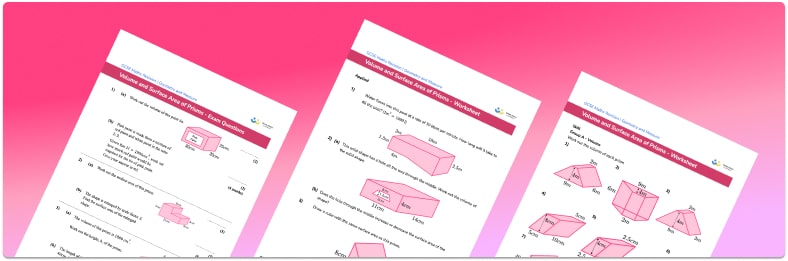# Surface Area And Volume Of Prisms WorksheetHelp your students prepare for their Maths GCSE with this free surface area and volume of prisms worksheet of 43 questions and answers

• Section 1 of the surface area and volume of prisms worksheet contains 36 skills-based surface area and volume of prisms questions, in 3 groups to support differentiation
• Section 2 contains 4 applied surface area and volume of prisms questions with a mix of word problems and deeper problem solving questions
• Section 3 contains 3 foundation and higher level GCSE exam style surface area and volume of prisms questions
• Answers and a mark scheme for all surface area and volume of prisms questions are provided
• Questions follow variation theory with plenty of opportunities for students to work independently at their own level
• All questions created by fully qualified expert secondary maths teachers
• Suitable for GCSE maths revision for AQA, OCR and Edexcel exam boards

• This field is for validation purposes and should be left unchanged.

You can unsubscribe at any time (each email we send will contain an easy way to unsubscribe). To find out more about how we use your data, see our privacy policy.

### Surface area and volume of prisms at a glance

A prism shape is a polyhedron (a 3D shape made from polygons) with a constant cross section through one dimension. A prism shape has two congruent faces (identical ends). Examples of prisms include rectangular prisms and triangular prisms. Shapes such as square pyramids are not prisms because the cross section is not constant throughout the shape.

To find the volume of a prism, we find the cross-sectional area and multiply it by the length. Volume is measured in units cubed.

To find the surface area of a prism, we find the area of each face and add the areas together. Surface area is measured in units squared.

To find the volume or surface area of 3d shapes which are not prisms we can use given formulae. For example, the formula for the surface area of a sphere is 4r2.

Looking forward, students can then progress to additional 3D shapes worksheets and more geometry worksheets for example an angles in polygons worksheet or how to calculate volume worksheet.For more teaching and learning support on Geometry our GCSE maths lessons provide step by step support for all GCSE maths concepts.

## Do you have KS4 students who need more focused attention to succeed at GCSE?There will be students in your class who require individual attention to help them succeed in their maths GCSEs. In a class of 30, it’s not always easy to provide.

Help your students feel confident with exam-style questions and the strategies they’ll need to answer them correctly with our dedicated GCSE maths revision programme.

Lessons are selected to provide support where each student needs it most, and specially-trained GCSE maths tutors adapt the pitch and pace of each lesson. This ensures a personalised revision programme that raises grades and boosts confidence.

Find out more